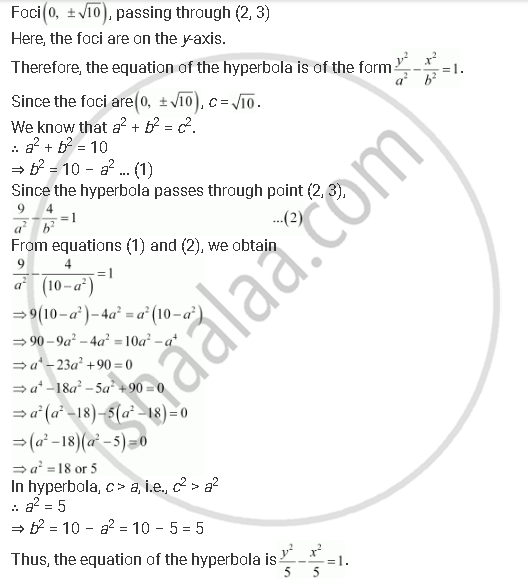# Find the Equation of the Hyperbola Satisfying the Give Conditions: Foci (0, +- Sqrt10), Passing Through (2, 3) - Mathematics

Find the equation of the hyperbola satisfying the give conditions: Foci (0, +- sqrt10), passing through (2, 3)

#### SolutionConcept: Hyperbola - Standard Equation of Hyperbola
Is there an error in this question or solution?

#### APPEARS IN

NCERT Class 11 Mathematics
Chapter 11 Conic Sections
Q 15 | Page 262